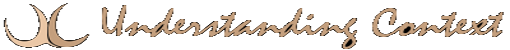# 26 Mar randomSomething that is devoid of a regular pattern. In the mathematical definition, it is random because we don’t yet understand the laws or formulas that govern it. In other definitions, it is due to its immunity to any law which would force it into a repeating sequence that would constitute a pattern. The existence of the lawless form of randomness is purely theoretical since there is no way to prove that anything is infinitely irregular or unpredictable. Small-scale quasi-randomness (as opposed to pure randomness) is a useful tool, particularly in game theory. Does the Copenhagen Interpretation prove that there is such a thing as randomness?

 Definitions References self-similarity    fractalchaos continuum    probabilityinference stochastic    chaoslogic dead man's hand probability

That’s so Random!

The Random Hamlet